## Example Questions

2 Next →

### Example Question #11 : How To Find The Whole From The Part With Percentageis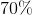of what number?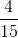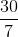Explanation:

We can set up a proportion to solve this problem. Remember that we can express percentages as fractions.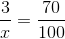Now we can cross-multiply and solve for our unknown.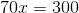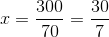2 Next →